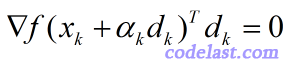# [原创] line search中的重要定理 - 梯度与方向的点积为零$\phi ({\alpha _k}) = f({x_k} + {\alpha _k}{d_k}) = \mathop {\min }\limits_{\alpha > 0} f({x_k} + \alpha {d_k}) = \mathop {\min }\limits_{\alpha > 0} \phi (\alpha )$

$\phi '({\alpha _k}) = {\left[ {f({x_k} + {\alpha _k}{d_k})} \right]^\prime } \cdot (0 + 1 \cdot {d_k}) = {\left[ {f({x_k} + {\alpha _k}{d_k})} \right]^\prime }{d_k} = \nabla f{({x_k} + {\alpha _k}{d_k})^T}{d_k} = 0$

➤➤ 版权声明 ➤➤